# How to select multiple columns in a pandas dataframe

• Difficulty Level : Easy
• Last Updated : 27 Nov, 2018

Python is a great language for doing data analysis, primarily because of the fantastic ecosystem of data-centric Python packages. Pandas is one of those packages and makes importing and analyzing data much easier.

Let’s discuss all different ways of selecting multiple columns in a pandas DataFrame.

Method #1: Basic Method

Given a dictionary which contains Employee entity as keys and list of those entity as values.

 `# Import pandas package``import` `pandas as pd`` ` `# Define a dictionary containing employee data``data ``=` `{``'Name'``:[``'Jai'``, ``'Princi'``, ``'Gaurav'``, ``'Anuj'``],``        ``'Age'``:[``27``, ``24``, ``22``, ``32``],``        ``'Address'``:[``'Delhi'``, ``'Kanpur'``, ``'Allahabad'``, ``'Kannauj'``],``        ``'Qualification'``:[``'Msc'``, ``'MA'``, ``'MCA'``, ``'Phd'``]}`` ` `# Convert the dictionary into DataFrame ``df ``=` `pd.DataFrame(data)`` ` `# select two columns``df[[``'Name'``, ``'Qualification'``]]`

Output: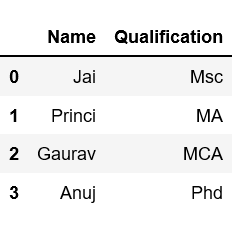Select Second to fourth column.

 `# Import pandas package``import` `pandas as pd`` ` `# Define a dictionary containing employee data``data ``=` `{``'Name'``:[``'Jai'``, ``'Princi'``, ``'Gaurav'``, ``'Anuj'``],``        ``'Age'``:[``27``, ``24``, ``22``, ``32``],``        ``'Address'``:[``'Delhi'``, ``'Kanpur'``, ``'Allahabad'``, ``'Kannauj'``],``        ``'Qualification'``:[``'Msc'``, ``'MA'``, ``'MCA'``, ``'Phd'``]}`` ` `# Convert the dictionary into DataFrame ``df ``=` `pd.DataFrame(data)`` ` `# select all rows ``# and second to fourth column``df[df.columns[``1``:``4``]]`

Output: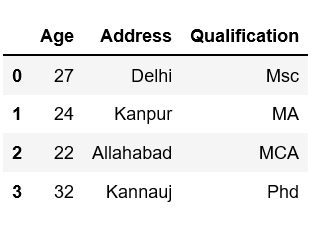Method #2: Using `loc[]`

Example 1: Select two columns

 `# Import pandas package``import` `pandas as pd`` ` `# Define a dictionary containing employee data``data ``=` `{``'Name'``:[``'Jai'``, ``'Princi'``, ``'Gaurav'``, ``'Anuj'``],``        ``'Age'``:[``27``, ``24``, ``22``, ``32``],``        ``'Address'``:[``'Delhi'``, ``'Kanpur'``, ``'Allahabad'``, ``'Kannauj'``],``        ``'Qualification'``:[``'Msc'``, ``'MA'``, ``'MCA'``, ``'Phd'``]}`` ` `# Convert the dictionary into DataFrame ``df ``=` `pd.DataFrame(data)`` ` `# select three rows and two columns``df.loc[``1``:``3``, [``'Name'``, ``'Qualification'``]]`

Output: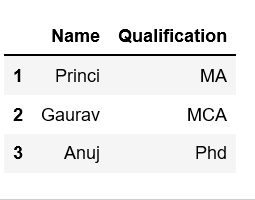Example 2: Select one to another columns. In our case we select column name “Name” to “Address”.

 `# Import pandas package``import` `pandas as pd`` ` `# Define a dictionary containing employee data``data ``=` `{``'Name'``:[``'Jai'``, ``'Princi'``, ``'Gaurav'``, ``'Anuj'``],``        ``'Age'``:[``27``, ``24``, ``22``, ``32``],``        ``'Address'``:[``'Delhi'``, ``'Kanpur'``, ``'Allahabad'``, ``'Kannauj'``],``        ``'Qualification'``:[``'Msc'``, ``'MA'``, ``'MCA'``, ``'Phd'``]}`` ` `# Convert the dictionary into DataFrame ``df ``=` `pd.DataFrame(data)`` ` `# select two rows and ``# column "name" to "Address"``# Means total three columns``df.loc[``0``:``1``, ``'Name'``:``'Address'``]`

Output: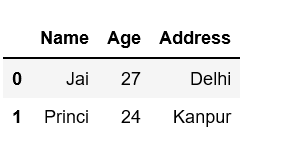Example 3: First filtering rows and selecting columns by label format and then Select all columns.

 `# Import pandas package``import` `pandas as pd`` ` `# Define a dictionary containing employee data``data ``=` `{``'Name'``:[``'Jai'``, ``'Princi'``, ``'Gaurav'``, ``'Anuj'``],``        ``'Age'``:[``27``, ``24``, ``22``, ``32``],``        ``'Address'``:[``'Delhi'``, ``'Kanpur'``, ``'Allahabad'``, ``'Kannauj'``],``        ``'Qualification'``:[``'Msc'``, ``'MA'``, ``'MCA'``, ``'Phd'``]``       ``}``# Convert the dictionary into DataFrame ``df ``=` `pd.DataFrame(data)`` ` `# .loc DataFrame method``# filtering rows and selecting columns by label``# format``# df.loc[rows, columns]``# row 1, all columns``df.loc[``0``, :]`

Output: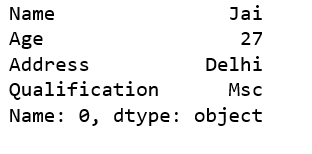Method #3: Using `iloc[]`

Example 1: Select first two column.

 `# Import pandas package``import` `pandas as pd`` ` `# Define a dictionary containing employee data``data ``=` `{``'Name'``:[``'Jai'``, ``'Princi'``, ``'Gaurav'``, ``'Anuj'``],``        ``'Age'``:[``27``, ``24``, ``22``, ``32``],``        ``'Address'``:[``'Delhi'``, ``'Kanpur'``, ``'Allahabad'``, ``'Kannauj'``],``        ``'Qualification'``:[``'Msc'``, ``'MA'``, ``'MCA'``, ``'Phd'``]}`` ` `# Convert the dictionary into DataFrame ``df ``=` `pd.DataFrame(data)`` ` `# Remember that Python does not``# slice inclusive of the ending index.``# select all rows ``# select first two column``df.iloc[:, ``0``:``2``] `

Output: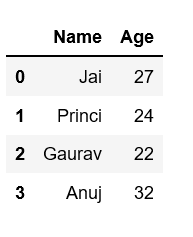Example 2: Select all or some columns, one to another using .iloc.

 `# Import pandas package``import` `pandas as pd`` ` `# Define a dictionary containing employee data``data ``=` `{``'Name'``:[``'Jai'``, ``'Princi'``, ``'Gaurav'``, ``'Anuj'``],``        ``'Age'``:[``27``, ``24``, ``22``, ``32``],``        ``'Address'``:[``'Delhi'``, ``'Kanpur'``, ``'Allahabad'``, ``'Kannauj'``],``        ``'Qualification'``:[``'Msc'``, ``'MA'``, ``'MCA'``, ``'Phd'``]}`` ` `# Convert the dictionary into DataFrame ``df ``=` `pd.DataFrame(data)`` ` `# iloc[row slicing, column slicing]``df.iloc [``0``:``2``, ``1``:``3``]`

Output: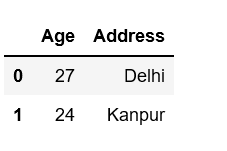Method #4: Using `.ix`

Select all or some columns, one to another using `.ix`.

 `# Import pandas package``import` `pandas as pd`` ` `# Define a dictionary containing employee data``data ``=` `{``'Name'``:[``'Jai'``, ``'Princi'``, ``'Gaurav'``, ``'Anuj'``],``        ``'Age'``:[``27``, ``24``, ``22``, ``32``],``        ``'Address'``:[``'Delhi'``, ``'Kanpur'``, ``'Allahabad'``, ``'Kannauj'``],``        ``'Qualification'``:[``'Msc'``, ``'MA'``, ``'MCA'``, ``'Phd'``]}`` ` `# Convert the dictionary into DataFrame ``df ``=` `pd.DataFrame(data)`` ` `# select all rows and 0 to 2 columns ``print``(df.ix[:, ``0``:``2``])`

Output: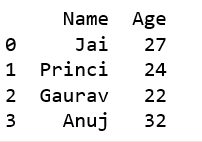My Personal Notes arrow_drop_up